• 如图 <p style="text-align:center"><img ... 不论怎么画都是一条直线，但明显这不是线性关系。不明白怎么控制这个y轴的刻度，去网上找了半天也没找到解决方案。求解答这是为什么，该怎么处理</p>python
• 最近又有朋友来询问为何他的时序数据用ARIMA模型后出现的是一条直线，像下面这样（截取部分数据显示）： 仔细看，阴影部分是预测后的结果走势，这里是蓝色的直线？？？ 是不是很奇怪，为何这里会产生一条直线？ ...
最近又有朋友（感谢这位朋友😁）来询问为何他的时序数据用ARIMA模型后出现的是一条直线，像下面这样（图源网络，仅作参考解释说明的示例）：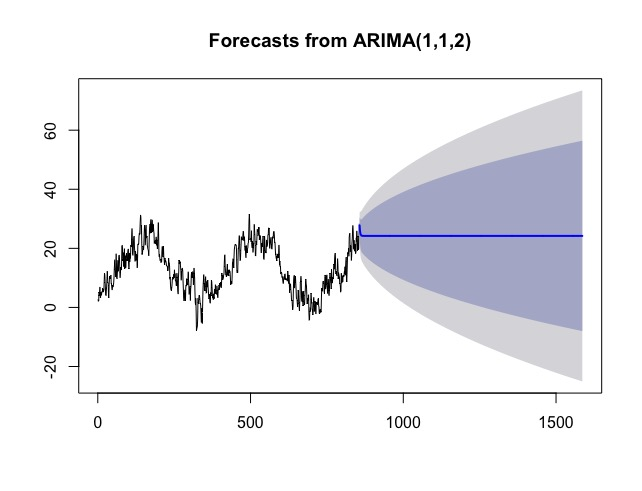是不是很奇怪，为何这里会产生一条直线？掌柜查阅了一番后发现原来是这么回事，下面进行讲解。
首先，使用ARIMA模型进行时序预测为何会出现是一条直线？  解答：简单来说是因为你的时序数据中存在“季节/周期性”（注：这里的季节性/周期性指的是 以同样的频率 持续重复出现）的特征。所以模型无法识别你的数据到底是哪种季节/周期特征（比如以“周”为单位的weekly季节/周期，或以“月”为单位的monthly季节/周期等），最后就只能输出一条直线来代替。  其次，如何确定时序数据中有“季节/周期性”特征存在的？？？  解答：
第一种方法：可以用statsmodels库里面的seasonal_decompose 函数来分解检测时序数据中的季节/周期性。具体方法如下：
import statsmodels.api as sm

#extrapolate_trend='freq'表示trend 、resid频率会从最近点开始，并且会对最近点的缺失值进行填充
#更多参数设置请参考官方文档：https://www.statsmodels.org/stable/generated/statsmodels.tsa.seasonal.seasonal_decompose.html
plt.rc('figure',figsize=(12,8))
fig = decomposition.plot()
plt.show()

最后得到的可视化分解图如下：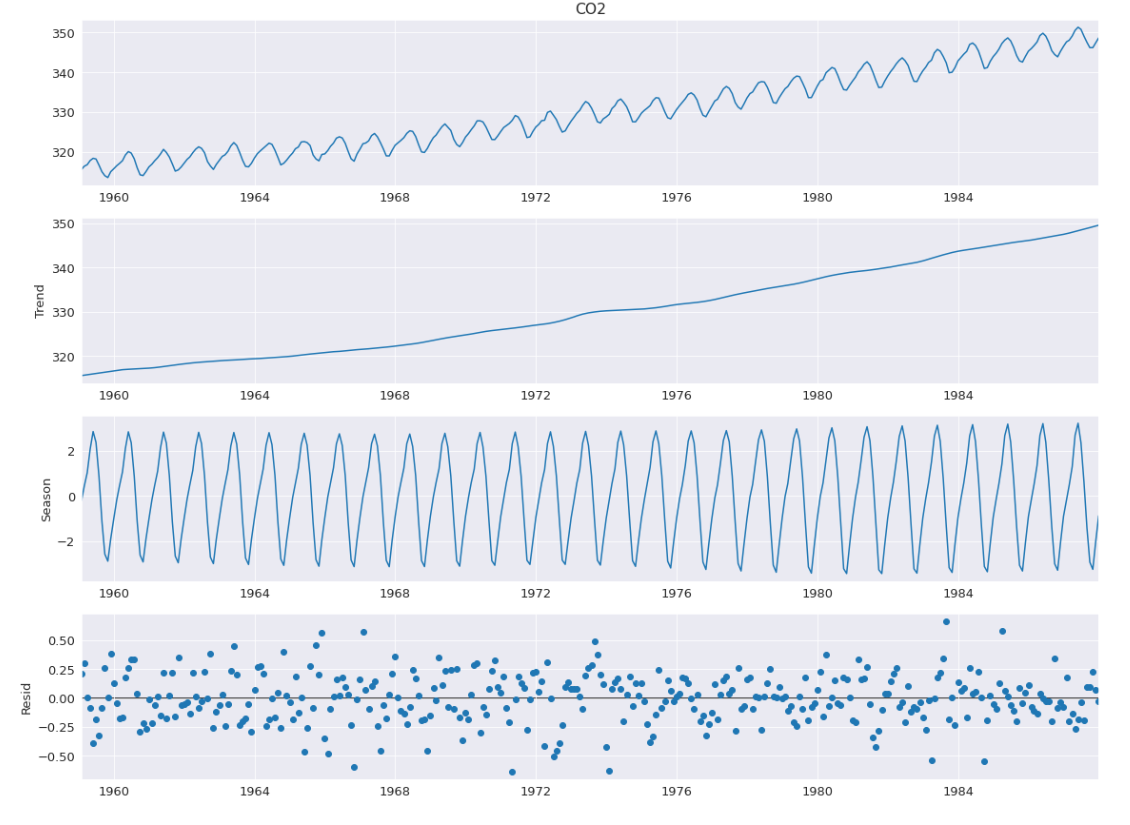可以明显的从第三个图Seasonal看出来，这个时序数据集确实是存在季节/周期性特征的。
同样用这个方法我们可以对比看，分解经典那篇的时序数据，最后得到的图片是这样的：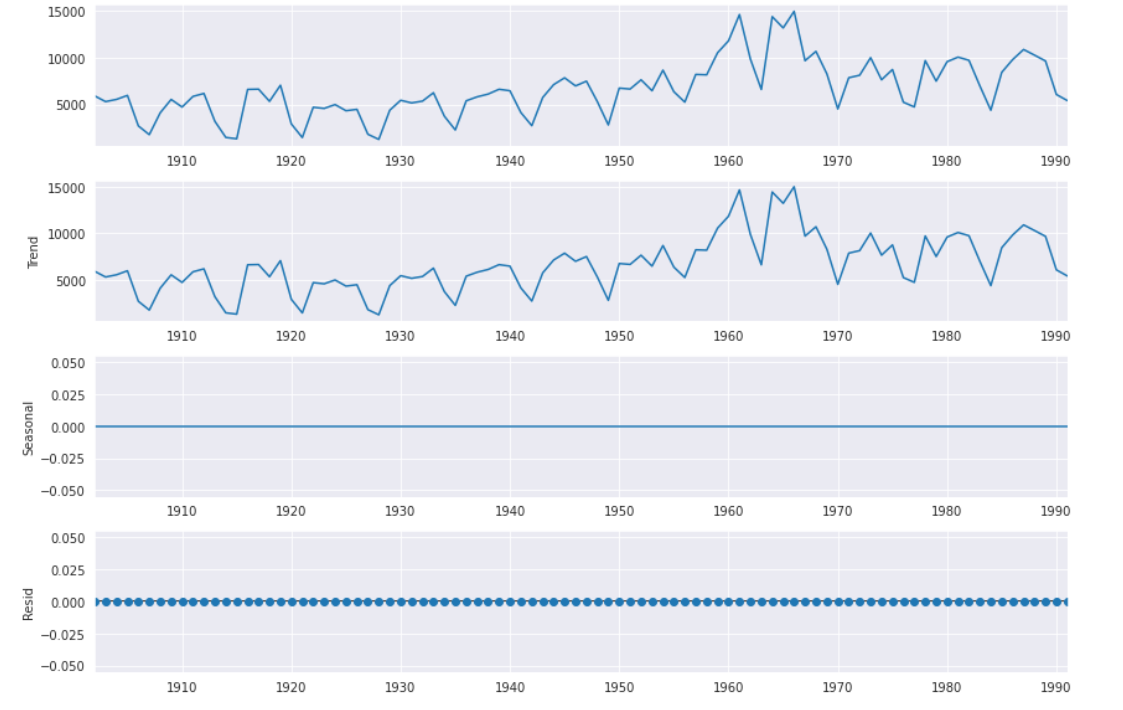第三个Seasonal是一条直线说明那段时序不具有 季节/周期性。
第二种方法：就是直接plot 来观察 时序图（对于季节/周期性很明显的情况，比如👇这图）。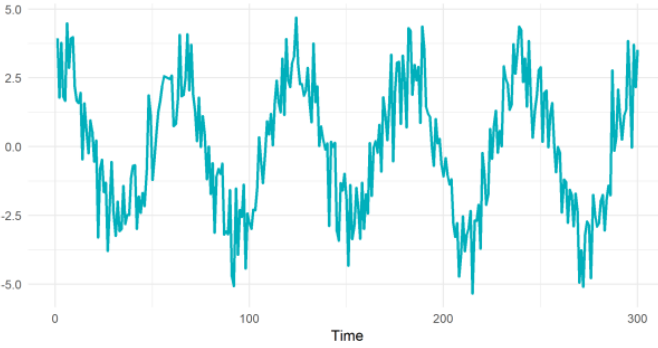（图源：网络）  第三种方法：FFT (全称叫做 Fast Fourier Transform)。是一种计算序列的离散傅里叶变换的算法，它将原始信号（这里是时间）转换为一定的频率来表示（说人话就是👉基于时间找到周期性变化的幅度、偏移量等因素）。不过该方法对多季节的时序数据效果不太好，这里不做演示。  最后，已知有季节/周期性数据存在，所以就不能再用那个简单的ARIMA模型来处理。
参考资料： ARIMA model producing a straight line prediction arima-forecast-straight-line Classical decomposition seasonal_decompose 函数的官方文档
展开全文数据分析 时间序列分析 ARIMA 时序分解
• 本文实例讲述了Python实现在matplotlib中两个坐标轴之间画一条直线光标的方法。分享给大家供大家参考。具体如下： 看看下面的例子和效果吧 # -*- coding: utf-8 -*- from matplotlib.widgets import MultiCursor ...
• import matplotlib.pyplot as plt import numpy x = numpy.linspace(-1,1,100) #从-...plt.plot(x,y) #命令行中出现[<matplotlib.lines.Line2D object at 0x111C04B0>] #但并没有画出图形 #运行下面这行后才出...
import matplotlib.pyplot as plt
import numpy
x = numpy.linspace(-1,1,100) #从-1到1生成100个点，用于横坐标
y = 2*x+1
plt.plot(x,y) #命令行中出现[<matplotlib.lines.Line2D object at 0x111C04B0>]
#但并没有画出图形
#运行下面这行后才出现图像
plt.show()

展开全文• 如何在已有图形上面添加一条直线？ 使用abline()函数。示例代码如下。 &gt;x &lt;- c(1.0, 2.0, 3.0, 4.0, 5.0) &gt;y &lt;- c(1.0, 1.9, 3.1, 4.0, 4.9) &gt;plot(x,y)&gt;abline...

如何在已有图形上面添加一条直线？

使用abline()函数。示例代码如下。

>x <- c(1.0, 2.0, 3.0, 4.0, 5.0)

>y <- c(1.0, 1.9, 3.1, 4.0, 4.9)

>plot(x,y)>abline(v=3,lwd=4,col="blue")#添加一条垂直直线x=3，线宽为4，颜色蓝色

>abline(h=3,lwd=4,col="blue")#添加一条水平直线y=3，线宽为4，颜色蓝色

>abline(lm(y~x), lwd=4, col="red")#添加一条一元线性回归拟合直线，线宽为4，颜色为红色


展开全文• 本博文源于matlab基础，每个图像个案例引入，大家简单看，直接照猫画虎去套用就行了。 例子：画y=2*x+3 范围为[1,10] 例子：在区间[-Π,Π]上，绘制函数y=sinx的图形 例子：画出椭圆(x*x)/25 + y^2/81 =1的曲线图...
本博文源于matlab基础，每个图像一个案例引入，大家简单看，直接照猫画虎去套用就行了。
画直线
例子：画y=2*x+3 范围为[1,10]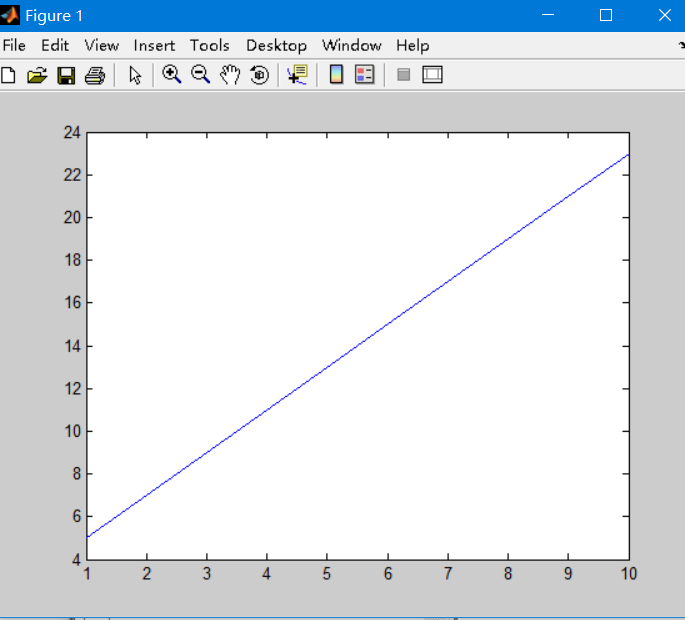代码：
>> x=1:10;
>> y=2*x+3;
>> plot(y)
>>

画曲线
在区间[-Π,Π]上，绘制函数y=sinx的图形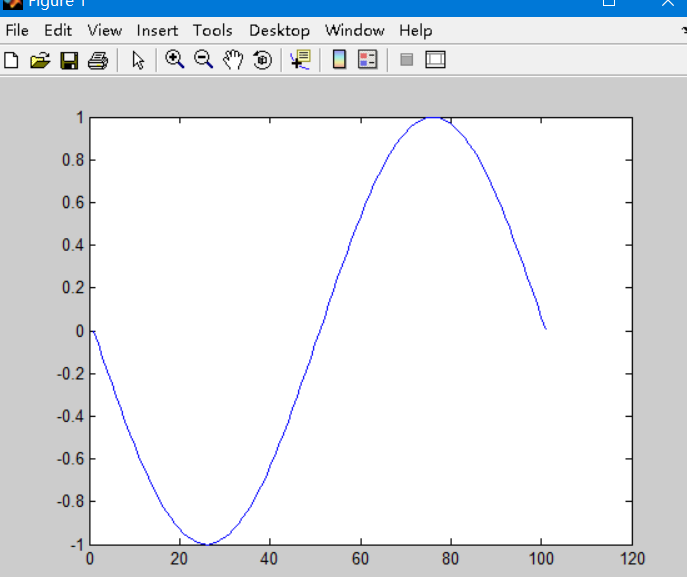>> x=-pi:pi/50:pi;
>> y=sin(x);
>> plot(y)
>>

画椭圆
例子：画出椭圆(xx)/25 + y^2/81 =1的曲线图，x属于[0,2pi]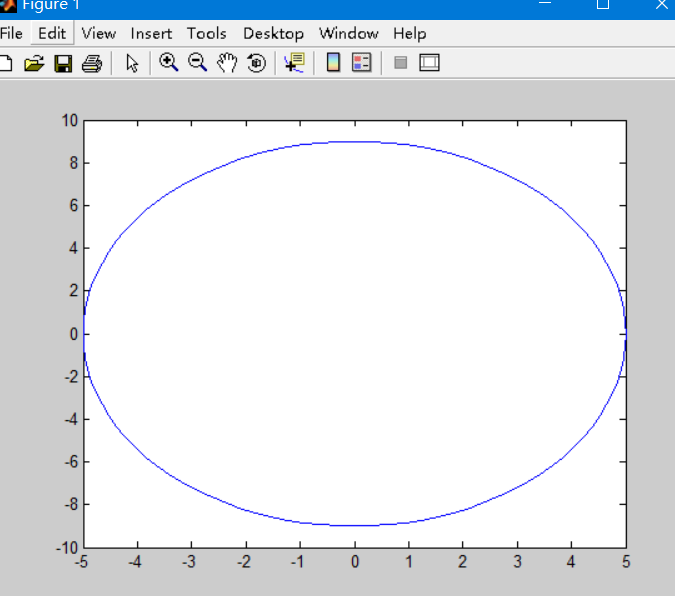展开全文matlab
• 怎么在matlab已经用plot画好的图中再加一条水平线 使用matlab画图，有时不知道绘制图片的范围，并且想要最后在这个图片上加一条水平线或者竖直线。怎么才能在matlab已经用plot画好的图中再加一条水平线并且不影响...
• When we plot a line with slope and intercept, we usually/traditionally position the axes at the middle of the graph. In the below code, we move the left and bottom spines to the center of the graph ...
• plot(x2,y2,'--'); hold on; set(gca,'xTick',[0:10:120]); set(gca,'ytick',[0:28:56]); values = spcrv([[x1(1) x1 x1(end)];[y1(1) y1 y1(end)]],3); plot(values(1,:),values(2,:), 'g'); title('实际温度和...matlab
• 参考网站：https://www.python-course.eu/dividing_lines_between_classes.php 上一章提到了一条直线分开了两个点，
• 如何在已有图形上面添加一条直线？ 使用abline()函数。示例代码如下。 >x <- c(1.0, 2.0, 3.0, 4.0, 5.0) >y <- c(1.0, 1.9, 3.1, 4.0, 4.9) >plot(x,y)>abline(v=3,lwd=4,col="blue")#...python .net go bug
• plt.plot(predictions,label='predictions') plt.plot(labels,label='real') plt.legend() plt.show() f=open('IBM.csv') df=pd.read_csv(f) seq=df.iloc[:,1].values train_data=seq[2:504] test_...tensorflow
• axline函数作用是绘制一条无限长的直线。与 axvline/axhline相比，axline函数绘制的直线长度是无限的，直线可以是任意角度的直线。 axline函数的签名为： matplotlib.pyplot.axline(xy1, xy2=None, *, slope=None, *...matplotlib pyplot
• 应用SVR对负荷数据进行回归分析，测试集出来的数为一条直线？ ` import numpy as np from sklearn.svm import SVR import pandas as pd import matplotlib.pyplot as plt import xlrd # 打开指定路径中的...tensorflow 人工智能 数据挖掘 神经网络
• import matplotlib.pyplot as plt plt.axhline(y=0.5, color='r', linestyle='-') plt.show() https://stackoverflow.com/questions/33382619/plot-a-horizontal-line-using-matplotlib
• 在matlab中，使用plot3函数可以通过两点绘制三维直线，语法如下： 设点A为（a1,a2,a3）,B为（b1,b2,b3） plot3([a1,b1],[a2,b2],[a3,b3]); 通过矩阵绘制起点为原点的向量举例如下： A = [ 1.0000000e-06 1.0000000e-...
•  % 画一条直线  x=rand*50 50; y=linspace;  I=bitmapplot;  % 画一条粗的红线  x=rand*50 100; y=linspace;  I=bitmapplot);  % 画一条带有标记的红线  x=rand*50 150; y=linspace;  I=...matlab
• matplotlib.pyplot.axhline https://matplotlib.org/stable/api/_as_gen/matplotlib.pyplot.axhline.html 用法： plt.axhline(0.06, color='r', linestyle='--', label='xxx') plt.legend(loc='upper left')
• 看看下面的例子和效果吧 # -*- coding: utf-8 -*- from matplotlib.widgets import MultiCursor ...下面是图形效果,看随着光标的移动,在两个图之间会画一条竖线   这个功能有时还是比较有用的matplotlib import 图形
• 这是一条斜率为样本标准差，截距为m的直线，就是在q-q图中代表着正态分布的直线。 在R语言中， q-q图还有95%置信区间， 哪位要是知道这两根虚线是怎么得到的，请不吝赐教，谢谢。R
• 开始是这样画的： import numpy as np #使用import导入模块numpy，并简写成np import matplotlib.pyplot as plt #使用import导入模块matplotlib.pyplot，并简写成plt plt.figure(figsize=(8,4)) #设置绘图
• 本博文源于matlab基础，主要讲解plot线条属性的使用。相信大家接触过matlab会明白，没接触过线条属性画图是这样子的：
• 直线 # 画出 x=2 这垂直线 plt.axvline(2) 点击此链接axvline查看帮助文档 水平线 # 画出 y=1 水平线 plt.axhline(1) 点击此链接axhline查看帮助文档python matplotlib 数据可视化 数据分析
• 您可以使用twiny()创建与条形轴共享yaxis的第二轴,并在该第二轴上绘制直线曲线.最困难的事情是如何对齐x轴刻度线.在这里,我们定义了align函数,它将ax2中的ax2.get_xlim()与x1对齐,而ax1中的ax2.get_xlim()...
• matplotlib之pyplot模块plot函数基础（函数功能、xy参数基本取值，多组数据）简单说明了plot函数绘制线条的基本功能。 plot函数的基本调用签名为plot([x], y, [fmt], *, data=None, **kwargs) 那么线条的外观该...matplotlib pyplot 格式字符串 fmt
• 最近学习TensorFlow，从最基本的做起，这是一个简单的拟合一条直线的例子，并且训练201次，每20步输出一次，并输出图像： 开始训练： 训练100步后： 以下是完整代码： #coding: utf-8 #author: 吴晶 #wechat...python tensorflow
• ## matlab中plot函数用法

万次阅读 多人点赞 2019-03-06 14:41:15
线条、颜色等参数： 1.简单的2维直线图 : plot(x,y) 同一坐标显示n条线：plot(x,y1,x,y2，…)...2.plot(X):X是矩阵，表示矩阵的每一行都画一条线，将显示n条线。 X=rand(3,3); %随机生成3*3矩阵 figure; hold on; p...matlab...